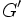# Finitely many commutators implies finite derived subgroup

This article gives a proof/explanation of the equivalence of multiple definitions for the term group with finite derived subgroup
View a complete list of pages giving proofs of equivalence of definitions

## Statement

Suppose$G$ is a group such that the set of commutators in$G$ is a finite set. Then, the derived subgroup of$G$ (i.e., the subgroup of$G$ generated by the set of commutators) is a finite group, and hence,$G$ is a group with finite derived subgroup.

## Caveats

Note that this statement is not saying that if the derived subgroup is finitely generated, then it is finite. That statement is in fact false -- the derived subgroup of the infinite dihedral group is an infinite cyclic group. The statement is really about a very specific choice of generating set for the derived subgroup, namely, the set of all commutators.

## Facts used

1. Finitely generated and FC implies FZ: The relevant part is that any finitely generated group that is also a FC-group (every conjugacy class is finite) is a FZ-group (the center has finite index).
2. FZ implies finite derived subgroup (this result is also called the Schur-Baer theorem).

## Proof

Given: A group$G$, with only finitely many elements that are commutators, say$g_1 = [h_1,k_1], g_2 = [h_2,k_2],\dots g_n = [h_n,k_n]$ (note that the$g_i$s are unique, but the$h_i,k_i$ are not uniquely determined).

To prove: The derived subgroup$G'$ of$G$ is finite.

Proof:

Step no. Assertion/construction Facts used Given data used Previous steps used Explanation
1 Let$H = \langle h_1,k_1,\dots,h_n,k_n \rangle$. Then,$H$ is a finitely generated subgroup of$G$ whose set of commutators is the same as the set of commutators of$G$, and it is a finite set. The whole given statement$H$ is finitely generated by definition. Since$H \le G$, every commutator of elements of$H$ is a commutator of elements of$G$. The other direction is obvious from the given: we've already written every commutator of elements of$G$ as a commutator of elements of$H$.
2$H$ is a FC-group, i.e., the size of the conjugacy class of every element$x \in H$ is finite. For any$y \in H$, we have$yxy^{-1} = yxy^{-1}x^{-1}x = [y,x]x$. Thus, every conjugate of$x$ is a commutator of elements of$H$ times the element$x$. Step (1) tells us that the set of commutators of elements of$H$ is finite, so the set of conjugates of$x$ is the image of a finite set and hence finite.
3$H$ is a FZ-group, i.e., the center of$H$ has finite index in$H$. Fact (1) Steps (1), (2) By Step (1),$H$ is finitely generated. By Step (2),$H$ is a FC-group. Thus, by Fact (1),$H$ is a FZ-group.
4 The derived subgroup$H'$ is finite. Fact (2) Step (3) Step-fact combination direct.
5$G'$ is finite. Steps (1), (4) Step (1) says that$G' = H'$. Combining with Step (4) gives the result.